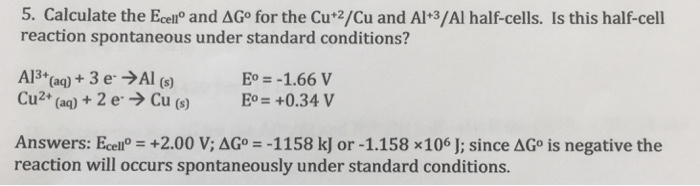# Question & Answer: Calculate the E_cell degree and delta G degree for the Cu^+2/Cu and Al^+3/Al half-cells. Is…..Calculate the E_cell degree and delta G degree for the Cu^+2/Cu and Al^+3/Al half-cells. Is this half-cell reaction spontaneous under standard conditions? Al^3+(aq) + 3 e^- rightarrow Al (s) E degree = -1.66 V Cu^2+(aq) + 2 e^- rightarrow Cu(s) E degree = + 0.34 V E_cell degree = + 2.00 V: delta G degree = – 1158 kJ or -1.158 kJ or -1.158 times 10^6 J: since delta G degree negative the reaction will occurs spontaneously under standard conditions.

Calculate the Ecell and delta G for the cu2+/cu and Al+32/Al half cells

E˚cell = E˚cathode – E˚anode

Don't use plagiarized sources. Get Your Custom Essay on
Question & Answer: Calculate the E_cell degree and delta G degree for the Cu^+2/Cu and Al^+3/Al half-cells. Is…..
GET AN ESSAY WRITTEN FOR YOU FROM AS LOW AS \$13/PAGE

E˚cell = 0.34 v – (-1.66 v) = +2.00 v——————-ANSWER

=======================================================================

ΔGo=−RTlnKeq=−nFEocell

=−nFEocell =

= (-6 mol e)(96458 C/mol e)(2.00 V)